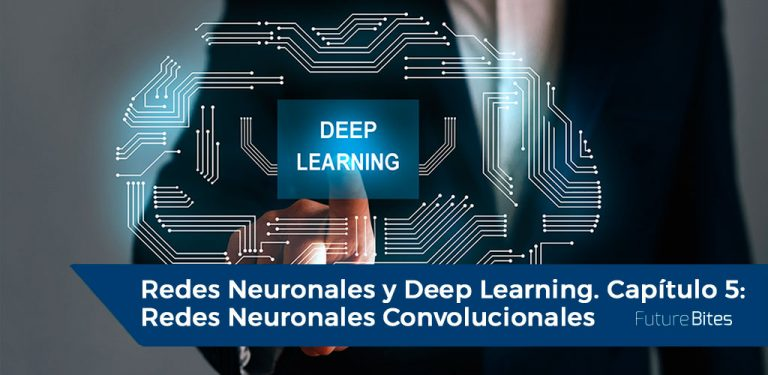# Neural Networks and Deep Learning. Chapter 5: Convolutional Neural NetworksUndoubtedly one of the most recurrent problems in the history of computer science is image processing and analysis. In other words, providing machines with "eyes" that enable them to understand the environment around them and make decisions based on the environment. This field of study is known as computer vision or artificial vision, and convolutional neural networks(CNNs in the following) are of vital importance in this field because they are specialists in working with images.

This type of network came to light in 1998 with an article by Yann LeCun, which laid the foundations for the intuitive idea that we will present below.

## How do convolutional neural networks work?

Well, we have said that convolutional neural networks are tremendously useful when working with images. Therefore, to understand how they work, it is useful to understand the logic of human vision, and that is what we will do next.

I'm pretty sure that all the readers have been able to recognise a car in the image above, but what if it was red instead of yellow? what if it was more modern? what if it was an SUV? In any case, the answer is that we will always be able to recognise a car, but why? Here's the kicker. Our brain has learned that there are a series of elements that make up a car: wheels, chassis, mirrors, headlights... and that they are always common. And further still, we can extend this logic recursively to each of the elements. For example, we have learned that a wheel is round and has a rim, that rear-view mirrors are mirrors that are positioned right next to the front doors...

This segmented learning is what an RNC tries to emulate. Moreover, it fits perfectly with the nature of the network itself, since as we saw in previous chapters a network has different layers that can be interpreted as stages of visual recognition. For example, in the first layers the network can focus on distinguishing geometric shapes, in the second layer it can associate circles to wheels, rectangles to glass... and in the last layers it can decide whether sufficient elements have been detected to classify the image as a car or not.

So much for the intuitive part, now we will get into the more technical part.

## The mathematics behind the RNCs

At this point we have to understand that an image, a priori, is nothing more than a binary matrix of pixels. In the matrix we will find a 1 in the positions where there are strokes and a 0 in those where there are not, as we can see below. Moreover, a matrix is easily "flattened" into a vector if we put for example all the rows one after the other. In this way we have converted an image into a vector, which is something consumable by an RNC.

It is worth mentioning that this is true when the image is in black and white. When it is a colour image the matrix is transformed into three, one corresponding to each of the RGB colours, but basically the idea would be the same.

However, if we look closely, the value of a pixel will always be closely related to that of its neighbouring pixel. This is where the concepts of convolution and filteringcome into play.

A convolution is nothing more than an operation that will generate a new matrix from a given one. To do this, it will apply a filter to the matrix that will basically generate a new pixel from the combination of mathematical operations between the original pixel and its nearest neighbours. In this way, if we move the filter all over our image, we can obtain a result like the ones shown below.

As we can see, there are many types of filter: to detect vertical edges, horizontal edges, to implement blurs... In fact, the values that this filter can take are infinite and each of them will contribute something different. But... Do we have to choose these values? The answer is no. As we saw in the previous chapter "Neural Networks and Deep Learning. Chapter 4: Backpropagation", we are able to give the network the autonomy to learn. In this case, this quality allows the network to learn which values and, in general, which filters are the most suitable for detecting features common to our classification object.

So much for mathematics today. In what follows we will deal with, among other topics, the most curious applications of these networks, don't miss it!

WE RECOMMEND YOU